# nrCDLChannel

Send signal through CDL channel model

Since R2018b

## Description

The `nrCDLChannel` System object™ sends an input signal through a clustered delay line (CDL) multi-input multi-output (MIMO) link-level fading channel to obtain the channel-impaired signal. The object implements the following aspects of TR 38.901 :

• Section 7.7.1: CDL models

• Section 7.7.3: Scaling of delays

• Section 7.7.5.1: Scaling of angles

• Section 7.7.6: K-factor for LOS channel models

To send a signal through the CDL MIMO channel model:

1. Create the `nrCDLChannel` object and set its properties.

2. Call the object with arguments, as if it were a function.

## Creation

### Syntax

``cdl = nrCDLChannel``
``cdl = nrCDLChannel(Name,Value)``

### Description

````cdl = nrCDLChannel` creates a CDL MIMO channel System object.```

example

````cdl = nrCDLChannel(Name,Value)` creates the object with properties set by using one or more name-value pairs. Enclose the property name inside quotes, followed by the specified value. Unspecified properties take default values.Example: ```cdl = nrCDLChannel('DelayProfile','CDL-D','DelaySpread',2e-6)``` creates the channel object with CDL-D delay profile and 2-microseconds delay spread.```

## Properties

expand all

Unless otherwise indicated, properties are nontunable, which means you cannot change their values after calling the object. Objects lock when you call them, and the `release` function unlocks them.

If a property is tunable, you can change its value at any time.

### Configurable Channel Properties

CDL delay profile, specified as `'CDL-A'`, `'CDL-B'`, `'CDL-C'`, `'CDL-D'`, `'CDL-E'`, or `'Custom'`. See TR 38.901 Section 7.7.1, Tables 7.7.1-1 to 7.7.1-5.

When you set this property to `'Custom'`, configure the delay profile using properties `PathDelays`, `AveragePathGains`, `AnglesAoA`, `AnglesAoD`, `AnglesZoA`, `AnglesZoD`, `HasLOSCluster`, `KFactorFirstCluster`, `AngleSpreads`, `XPR`, and `NumStrongestClusters`.

Data Types: `char` | `string`

Discrete path delays in seconds, specified as a numeric scalar or row vector. `AveragePathGains` and `PathDelays` must have the same size.

#### Dependencies

To enable this property, set `DelayProfile` to `'Custom'`.

Data Types: `double`

Average path gains in dB, also referred to as cluster powers in TR 38.901, specified as a numeric scalar or row vector. `AveragePathGains` and `PathDelays` must have the same size.

#### Dependencies

To enable this property, set `DelayProfile` to `'Custom'`.

Data Types: `double`

Azimuth of arrival angle in degrees, specified as a numeric scalar or row vector. The vector elements specify the angles for each cluster.

#### Dependencies

To enable this property, set `DelayProfile` to `'Custom'`.

Data Types: `double`

Azimuth of departure angle in degrees, specified as a numeric scalar or row vector. The vector elements specify the angles for each cluster.

#### Dependencies

To enable this property, set `DelayProfile` to `'Custom'`.

Data Types: `double`

Zenith of arrival angle in degrees, specified as a numeric scalar or row vector. The vector elements specify the angles for each cluster.

#### Dependencies

To enable this property, set `DelayProfile` to `'Custom'`.

Data Types: `double`

Zenith of departure angle in degrees, specified as a numeric scalar or row vector. The vector elements specify the angles for each cluster.

#### Dependencies

To enable this property, set `DelayProfile` to `'Custom'`.

Data Types: `double`

Line of sight (LOS) cluster of the delay profile, specified as `false` or `true`. The `PathDelays`, `AveragePathGains`, `AnglesAoA`, `AnglesAoD`, `AnglesZoA`, and `AnglesZoD` properties define the delay profile. To enable the LOS cluster of the delay profile, set `HasLOSCluster` to `true`.

#### Dependencies

To enable this property, set `DelayProfile` to `'Custom'`.

Data Types: `logical`

K-factor in the first cluster of the delay profile in dB, specified as a numeric scalar. The default value corresponds to the K-factor in the first cluster of CDL-D as defined in TR 38.901 Section 7.7.1, Table 7.7.1-4.

#### Dependencies

To enable this property, set `DelayProfile` to `'Custom'` and `HasLOSCluster` to `true`.

Data Types: `double`

Apply scaling of angles, specified as `false` or `true` according to TR 38.901 Section 7.7.5.1. When set to `true`, the `AngleSpreads` and `MeanAngles` properties define the scaling of angles.

#### Dependencies

To enable this property, set `DelayProfile` to `'CDL-A'`, `'CDL-B'`, `'CDL-C'`, `'CDL-D'`, or `'CDL-E'`. This property does not apply for custom delay profile.

Data Types: `logical`

Scaled or cluster-wise root mean square (RMS) angle spreads in degrees, specified as a four-element row vector in one of these forms:

• [ASD ASA ZSD ZSA] — Use this vector to specify the desired RMS angle spreads of the channel, as described in TR 38.901 Section 7.7.5.1 (ASdesired), where:

• ASD is the RMS azimuth spread of departure angles

• ASA is the RMS azimuth spread of arrival angles

• ZSD is the RMS zenith spread of departure angles

• ZSA is the RMS zenith spread of arrival angles

To use this form, set `AngleScaling` to `true` and `DelayProfile` to `'CDL-A'`, `'CDL-B'`, `'CDL-C'`, `'CDL-D'`, or `'CDL-E'`.

• [CASD CASA CZSD CZSA] — Use this vector to specify cluster-wise RMS angle spreads for scaling ray offset angles within a cluster, as described in TR 38.901 Section 7.7.1, Step1, where:

• CASD is the cluster-wise RMS azimuth spread of departure angles

• CASA is the cluster-wise RMS azimuth spread of arrival angles

• CZSD is the cluster-wise RMS zenith spread of departure angles

• CZSA is the cluster-wise RMS zenith spread of arrival angles

To use this form, set `DelayProfile` to `'Custom'`. Based on TR 38.901 Section 7.7.5.1, the object does not perform angle scaling in this case.

The default value corresponds to the default cluster-wise angle spreads of CDL-A as defined in TR 38.901 Section 7.7.1 Table 7.7.1-1.

#### Dependencies

To enable this property, set `DelayProfile` to `'Custom'` or `AngleScaling` to `true`.

Data Types: `double`

Scaled mean angles in degrees, specified as a four-element row vector of the form [AoD AoA ZoD ZoA].

• AoD is the mean azimuth of departure angles after scaling

• AoA is the mean azimuth of arrival angles after scaling

• ZoD is the mean zenith of departure angles after scaling

• ZoA is the mean zenith of arrival angles after scaling

Use this vector to specify the desired mean angles of the channel used for angle scaling, as described in TR 38.901 Section 7.7.5.1 (${\mu }_{\Phi ,\text{desired}}$).

#### Dependencies

To enable this property, set `AngleScaling` to `true`.

Data Types: `double`

Coupling of departure and arrival rays within a cluster for azimuth and elevation, specified as one of these values.

• `'Random'` — The object randomly couples the rays, as defined in TR 38.901 Section 7.5 Step 8, using the random number stream specified by the `RandomStream` property.

• N-by-M-by-3 numeric array — Use this array to explicitly define the ray coupling. N is the number of clusters, equal to the number of path delays, specified by the `PathDelays` property. M is the number of rays per cluster, equal to 20. The three N-by-M planes, in the third dimension, correspond to the AoD/AoA, ZoD/ZoA, and AoD/ZoD ray couplings, respectively. Each row in each N-by-M plane specifies the ray coupling within the corresponding cluster by using a permutation of ray indices from 1 to M.

Note

#### Dependencies

To enable this property, set `DelayProfile` to `'Custom'`.

Data Types: `double` | `char` | `string`

Cross-polarization power ratio in dB, specified as a numeric scalar or an N-by-M numeric matrix. N is the number of clusters, equal to the number of path delays, specified by the `PathDelays` property. M is the number of rays per cluster, equal to 20. The default value corresponds to the cluster-wise cross-polarization power ratio of CDL-A as defined in TR 38.901 Section 7.7.1, Table 7.7.1-1.

Note

#### Dependencies

To enable this property, set `DelayProfile` to `'Custom'`.

Data Types: `double`

Initial phases of all rays for the four polarization combinations in degrees, specified as one of these values.

• `'Random'` — The object draws uniformly distributed random phases, as defined in TR 38.901 Section 7.5 Step 10, using the random number stream specified by the `RandomStream` property.

• N-by-M-by-4 numeric array — Use this option to explicitly define the initial phases. N is the number of clusters, equal to the number of path delays, specified by the `PathDelays` property. M is the number of rays per cluster, equal to 20. The four N-by-M planes, in the third dimension, correspond to the θ/θ, θ/ϕ, ϕ/θ, ϕ/ϕ polarization combinations, respectively.

Note

#### Dependencies

To enable this property, set `DelayProfile` to `'Custom'`.

Data Types: `double` | `char` | `string`

Desired RMS delay spread in seconds, specified as a numeric scalar. For examples of desired RMS delay spreads, `DSdesired`, see TR 38.901 Section 7.7.3 and Tables 7.7.3-1 and 7.7.3-2.

#### Dependencies

To enable this property, set `DelayProfile` to `'CDL-A'`, `'CDL-B'`, `'CDL-C'`, `'CDL-D'`, or `'CDL-E'`. This property does not apply for custom delay profile.

Data Types: `double`

Carrier frequency in Hz, specified as a numeric scalar.

Data Types: `double`

Maximum Doppler shift in Hz, specified as a nonnegative numeric scalar. This property applies to all channel paths. When the maximum Doppler shift is set to 0, the channel remains static for the entire input. To generate a new channel realization, reset the object by calling the `reset` function.

Data Types: `double`

User terminal (or user equipment) direction of travel in degrees, specified as a two-element column vector. The vector elements specify the azimuth and the zenith components [azimuth; zenith].

Data Types: `double`

K-factor scaling, specified as `false` or `true`. When set to `true`, the `KFactor` property specifies the desired K-factor and the object applies K-factor scaling as described in TR 38.901 Section 7.7.6.

Note

K-factor scaling modifies both the path delays and path powers.

#### Dependencies

To enable this property, set `DelayProfile` to `'CDL-D'` or `'CDL-E'`.

Data Types: `double`

Desired K-factor for scaling in dB, specified as a numeric scalar. For typical K-factor values, see TR 38.901 Section 7.7.6 and Table 7.5-6.

Note

• K-factor scaling modifies both the path delays and path powers.

• `K-factor` applies to the overall delay profile. Specifically, the K-factor before the scaling is `Kmodel`, as described in TR 38.901 Section 7.7.6. `Kmodel` is the ratio of the power of the first path LOS to the total power of all the Laplacian clusters, including the Laplacian part of the first cluster.

#### Dependencies

To enable this property, set `KFactorScaling` to `true`.

Data Types: `double`

Sample rate of the input signal in Hz, specified as a positive numeric scalar.

Data Types: `double`

Transmit antenna array characteristics, specified as a structure or a phased array (requires Phased Array System Toolbox™).

Phased arrays enable you to specify different antenna array configurations, including predefined and custom antenna elements. You can design custom antenna elements by using Phased Array System Toolbox or Antenna Toolbox™ features. To specify custom antenna elements in a 5G rectangular multipanel array, as defined in TR 38.901 Section 7.3, use the `phased.NRRectangularPanelArray` (Phased Array System Toolbox) object. For an overview of phased arrays, see Array Geometries and Analysis (Phased Array System Toolbox).

When specified as a structure, this property contains these fields:

Parameter FieldValuesDescription
`Size`

`[2 2 2 1 1]` (default),

row vector

Size of antenna array [M N P Mg Ng], where:

• M and N are the number of rows and columns in the antenna array, respectively.

• P is the number of polarizations (1 or 2).

• Mg and Ng are the number of row and column array panels, respectively.

The `nrCDLChannel` System object maps the input signal `signalIn` to the antenna array elements panel-wise, in the order that a 5-D array of size M-by-N-by-P-by-Mg-by-Ng is linearly indexed across the first dimension to the last.

For example, this figure shows how the object maps the input signal `signalIn` to an antenna array of size `[2 3 2 2 2]`. The antenna array consists of 2-by-2 antenna panels of 2-by-3 elements with 2 polarizations. The object maps the first M = 2 columns of the input signal (s1 and s2) to the first column of antenna elements with the first polarization angle of the first panel. The next M = 2 columns of the input signal (s3 and s4) are mapped to the next column of antenna elements, and so on. Following this pattern, the object maps the first M × N = 6 columns of the input signal (s1 to s6) to the antenna elements with the first polarization angle of the complete first panel. Similarly, the next 6 columns of the input signal (s7 to s12) are mapped to the antenna elements with the second polarization angle of the first panel. Subsequent sets of M × N × P = 12 columns of the input signal (s13 to s24, s25 to s36, s37 to s48) are mapped to consecutive panels, taking panel rows first, then panel columns.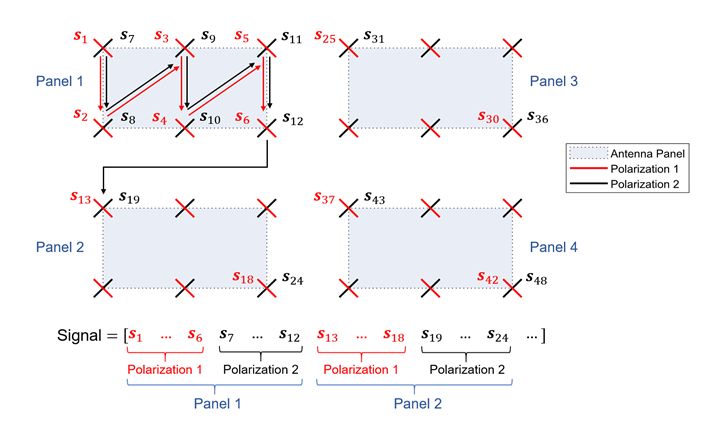`ElementSpacing`

`[0.5 0.5 1.0 1.0]` (default),

row vector

Element spacing, in wavelengths, specified as a row vector of the form [λv λh dgv dgh]. The vector elements represent the vertical and horizontal element spacing and the vertical and horizontal panel spacing, respectively. The panel spacing is measured from the center of the panels.

`PolarizationAngles`

`[45 -45]` (default),

row vector

Polarization angles in degrees, specified as a row vector of the form [θ ρ].

`Orientation` (to be removed)

`[0; 0; 0]`(default),

column vector

Note

This field will be removed in a future release. Use the `TransmitArrayOrientation` property instead.

Mechanical orientation of the array, in degrees, specified as a column vector of the form [α; β; γ]. The vector elements specify the bearing, downtilt, and slant, respectively. The default value indicates that the broadside direction of the array points to the positive x-axis.

`Element`

`'38.901'` (default),

`'isotropic'`

Antenna element radiation pattern as described in TR 38.901 Section 7.3. (Note that TR 38.901 superseded TR 38.900.)

`PolarizationModel`

```'Model-2' ```(default),

`'Model-1'`

Mechanical orientation of the transmit antenna array, specified as a three-element numeric column vector of the form [α; β; γ]. The vector elements specify the bearing, downtilt, and slant rotation angles in degrees, respectively, as specified in TR 38.901 Section 7.1.3. The object applies these rotation angles relative to the default array orientation in the local coordinate system. The default array orientation, corresponding to the value `[0; 0; 0]`, depends on the `TransmitAntennaArray` property.

• If you specify the `TransmitAntennaArray` property as a structure (default), in the default array orientation, the broadside direction points to the positive x-axis.

• If you specify the `TransmitAntennaArray` property as a phased array (requires Phased Array System Toolbox), you can configure the default array orientation by setting the relevant array properties of the specified phased array object.

To visualize and evaluate the resulting array orientation, call the `displayChannel` function on the `nrCDLChannel` channel model.

For an example of orienting transmit and receive antennas towards each other, see Orient Transmit and Receive Antennas Using LOS Path Angles.

Data Types: `double`

Receive antenna array characteristics, specified as a structure or a phased array (requires Phased Array System Toolbox).

Phased arrays enable you to specify different antenna array configurations, including predefined and custom antenna elements. You can design custom antenna elements by using Phased Array System Toolbox or Antenna Toolbox features. To specify custom antenna elements in a 5G rectangular multipanel array, as defined in TR 38.901 Section 7.3, use the `phased.NRRectangularPanelArray` (Phased Array System Toolbox) object. For an overview of phased arrays, see Array Geometries and Analysis (Phased Array System Toolbox).

When specified as a structure, this property contains these fields:

Parameter FieldValuesDescription
`Size`

`[1 1 2 1 1]` (default),

row vector

Size of antenna array [M N P Mg Ng], where:

• M and N are the number of rows and columns in the antenna array, respectively.

• P is the number of polarizations (1 or 2).

• Mg and Ng are the number of row and column array panels, respectively.

The `nrCDLChannel` System object maps the antenna array elements to the output signal `signalOut` panel-wise, in the order that a 5-D array of size M-by-N-by-P-by-Mg-by-Ng is linearly indexed across the first dimension to the last.

For example, this figure shows how the object maps an antenna array of size `[2 3 2 2 2]` to the output signal `signalOut`. The antenna array consists of 2-by-2 antenna panels of 2-by-3 elements with 2 polarizations. The first column of antenna elements with the first polarization angle of the first panel are mapped to the first M = 2 columns of the output signal (s1 and s2). The next column of antenna elements are mapped to the next M = 2 columns of the output signal (s3 and s4), and so on. Following this pattern, the object maps the antenna elements with the first polarization angle of the complete first panel to the first M × N = 6 columns of the output signal (s1 to s6). Similarly, the antenna elements with the second polarization angle of the first panel are mapped to the next 6 columns of the output signal (s7 to s12). Consecutive panels are mapped to subsequent sets of M × N × P = 12 columns of the output signal (s13 to s24, s25 to s36, s37 to s48), taking panel rows first, then panel columns.`ElementSpacing`

`[0.5 0.5 0.5 0.5]` (default),

row vector

Element spacing, in wavelengths, specified as a row vector of the form [λv λh dgv dgh]. The vector elements represent the vertical and horizontal element spacing and the vertical and horizontal panel spacing, respectively. The panel spacing is measured from the center of the panels.

`PolarizationAngles`

`[0 90]` (default),

row vector

Polarization angles in degrees, specified as a row vector of the form [θ ρ].

`Orientation` (to be removed)

`[0; 0; 0]`(default),

column vector

Note

This field will be removed in a future release. Use the `ReceiveArrayOrientation` property instead.

Mechanical orientation of the array, in degrees, specified as a column vector of the form [α; β; γ]. The vector elements specify the bearing, downtilt, and slant, respectively. The default value indicates that the broadside direction of the array points to the positive x-axis.

`Element`

`'isotropic'` (default),

`'38.901'`

Antenna element radiation pattern as described in TR 38.901 Section 7.3. (Note that TR 38.901 superseded TR 38.900.)

`PolarizationModel`

`'Model-2' `(default),

`'Model-1'`

Mechanical orientation of the receive antenna array, specified as a three-element numeric column vector of the form [α; β; γ]. The vector elements specify the bearing, downtilt, and slant rotation angles in degrees, respectively, as specified in TR 38.901 Section 7.1.3. The object applies these rotation angles relative to the default array orientation in the local coordinate system. The default array orientation, corresponding to the value `[0; 0; 0]`, depends on the `ReceiveAntennaArray` property.

• If you specify the `ReceiveAntennaArray` property as a structure (default), in the default array orientation, the broadside direction points to the positive x-axis.

• If you specify the `ReceiveAntennaArray` property as a phased array (requires Phased Array System Toolbox), you can configure the default array orientation by setting the relevant array properties of the specified phased array object.

To visualize and evaluate the resulting array orientation, call the `displayChannel` function on the `nrCDLChannel` channel model.

For an example of orienting transmit and receive antennas towards each other, see Orient Transmit and Receive Antennas Using LOS Path Angles.

Data Types: `double`

Number of time samples per half wavelength, specified as `Inf` or a numeric scalar. The `SampleDensity` and `MaximumDopplerShift` properties control the coefficient generation sampling rate, Fcg, given by

Fcg = `MaximumDopplerShift` × 2 × `SampleDensity`.

Setting `SampleDensity` to `Inf` assigns Fcg the value of the `SampleRate` property.

For an example of how sample density affects the channel output and path gains, see Explore the Effect of SampleDensity Property in CDL Channel Output.

Data Types: `double`

Normalized channel fading process, specified as `true` or `false`. When this property is set to `true`, the amplitude of the channel fading process is normalized by the average path gains (also referred to as cluster powers in TR 38.901). This normalization does not include other channel gains, for example, polarization and antenna element directivity. When this property is set to `false`, the channel fading process is not normalized. The `DelayProfile` property determines the average path gains, based on TR 38.901 Section 7.7.1, Tables 7.7.1-1 to 7.7.1-5. When you set `DelayProfile` to `'Custom'`, you can specify the average path gains with the `AveragePathGains` property.

Data Types: `logical`

Time offset of fading process in seconds, specified as a numeric scalar.

Tunable: Yes

Data Types: `double`

Number of strongest clusters to split into subclusters, specified as a numeric scalar. See TR 38.901 Section 7.5, Step 11.

#### Dependencies

To enable this property, set `DelayProfile` to `'Custom'`.

Data Types: `double`

Cluster delay spread in seconds, specified as a nonnegative scalar. Use this property to specify the delay offset between subclusters for clusters split into subclusters. See TR 38.901 Section 7.5, Step 11.

#### Dependencies

To enable this property, set `DelayProfile` to `'Custom'` and `NumStrongestClusters` to a value greater than zero.

Data Types: `double`

Source of the random number stream to initialize the ray phases and coupling using uniformly distributed random numbers, specified as one of these values.

• `'mt19937ar with seed'` — The object uses the mt19937ar algorithm for the random number generation. Calling the `reset` function resets the filters and reinitializes the random number stream to the value of the `Seed` property. Specifying this value results in repeatable channel fading.

• `'Global stream'` — The object uses the current global random number stream for the random number generation. Calling the `reset` function resets only the filters.

#### Dependencies

To enable this property, set the `RayCoupling` or `InitialPhases` properties to `'Random'`.

Initial seed of mt19937ar random number stream, specified as a nonnegative numeric scalar.

#### Dependencies

To enable this property, set RandomStream to `'mt19937ar with seed'`. When calling the `reset` function, the seed reinitializes the mt19937ar random number stream.

Data Types: `double`

Normalize channel outputs, specified as `true` or `false`. When this property is set to `true`, the channel output signal is divided by the square-root of the number of receive antennas. The normalization is by NR, where NR is the number of receive antenna elements or the number of antenna subarrays (only when you specify the `ReceiveAntennaArray` property as a `phased.ReplicatedSubarray` (Phased Array System Toolbox) or `phased.PartitionedArray` (Phased Array System Toolbox) phased array object). To determine the value of NR, check the `NumOutputSignals` structure field in the output of the `info(cdl)` object function call.

Note

When you call the `swapTransmitAndReceive` function to reverse the role of the transmit and receive antennas within the channel, the function also swaps these output structure fields of the `info(cdl)` function call:

• `NumTransmitAntennas` and `NumReceiveAntennas`

• `NumInputSignals` and `NumOutputSignals`

Hence the normalization is always by NR.

#### Dependencies

To enable this property, set `ChannelFiltering` to `true`.

Data Types: `logical`

Fading channel filtering, specified as `true` or `false`. When this property is set to `false`, these conditions apply.

For a use case of disabling channel filtering, see the CDL Channel Model Customization with Ray Tracing example.

Data Types: `logical`

Number of time samples, specified as a positive integer. Use this property to set the duration of the fading process realization.

Tunable: Yes

#### Dependencies

To enable this property, set `ChannelFiltering` to `false`.

Data Types: `double`

Data type of generated path gains, specified as `'double'` or `'single'`.

#### Dependencies

To enable this property, set `ChannelFiltering` to `false`.

Data Types: `double`

### Nonconfigurable Channel Properties

Reversed channel link direction, returned as one of these values.

• `false` — The role of the transmit and receive antennas within the channel model corresponds to the original channel link direction. Calling the `swapTransmitAndReceive` function on the `nrCDLChannel` object reverses the link direction of the channel and toggles this property value from `false` to `true`.

• `true` — The role of the transmit and receive antennas within the channel model are swapped. Calling the `swapTransmitAndReceive` function on the `nrCDLChannel` object restores the original link direction of the channel and toggles this property value from `true` to `false`.

Data Types: `logical`

## Usage

### Syntax

``signalOut = cdl(signalIn)``
``[signalOut,pathGains] = cdl(signalIn)``
``[signalOut,pathGains,sampleTimes] = cdl(signalIn)``
``[pathGains,sampleTimes] = cdl()``

### Description

example

````signalOut = cdl(signalIn)` sends the input signal through a CDL MIMO fading channel and returns the channel-impaired signal.```
````[signalOut,pathGains] = cdl(signalIn)` also returns the MIMO channel path gains of the underlying fading process.```

example

````[signalOut,pathGains,sampleTimes] = cdl(signalIn)` also returns the sample times of the channel snapshots of `pathGains` (first-dimension elements).```
````[pathGains,sampleTimes] = cdl()` returns only the path gains and the sample times. The `cdl` object acts as a source of the path gains and sample times without filtering an input signal. The `NumTimeSamples` object property specifies the duration of the fading process and the `OutputDataType` object property specifies the data type of the generated path gains. To use this syntax, you must set the `ChannelFiltering` object property to `false`.```

### Input Arguments

expand all

Input signal, specified as a complex scalar, column vector, or NS-by-NT matrix, where:

Data Types: `single` | `double`
Complex Number Support: Yes

### Output Arguments

expand all

Output signal, returned as a complex scalar, vector, or NS-by-NR matrix, where:

The output signal data type is of the same precision as the input signal data type.

Data Types: `single` | `double`
Complex Number Support: Yes

MIMO channel path gains of the fading process, returned as an NCS-by-NP-by-NT-by-NR complex array, where:

The path gains data type is of the same precision as the input signal data type.

Data Types: `single` | `double`
Complex Number Support: Yes

Sample times of channel snapshots, returned as an NCS-by-1 column vector, where NCS is the number of channel snapshots controlled by the `SampleDensity` property.

Data Types: `double`

## Object Functions

To use an object function, specify the System object as the first input argument. For example, to release system resources of a System object named `obj`, use this syntax:

`release(obj)`

expand all

 `info` Characteristic information of link-level MIMO channel `getPathFilters` Get path filter impulse response for link-level MIMO channel `displayChannel` Visualize and explore CDL channel model characteristics `swapTransmitAndReceive` Reverse link direction in CDL channel model
 `step` Run System object algorithm `clone` Create duplicate System object `isLocked` Determine if System object is in use `release` Release resources and allow changes to System object property values and input characteristics `reset` Reset internal states of System object

## Examples

collapse all

Transmit waveform through a clustered delay line (CDL) channel model with delay profile CDL-D from TR 38.901 Section 7.7.1.

Define the channel configuration structure using an `nrCDLChannel` System object. Use delay profile CDL-D, a delay spread of 10 ns, and UE velocity of 15 km/h:

```v = 15.0; % UE velocity in km/h fc = 4e9; % carrier frequency in Hz c = physconst('lightspeed'); % speed of light in m/s fd = (v*1000/3600)/c*fc; % UE max Doppler frequency in Hz cdl = nrCDLChannel; cdl.DelayProfile = 'CDL-D'; cdl.DelaySpread = 10e-9; cdl.CarrierFrequency = fc; cdl.MaximumDopplerShift = fd;```

Configure the transmit array layout as a vector of the form [M N P Mg Ng] = [2 4 2 1 2], representing 2 panels (Mg=1, Ng=2) with a 2-by-4 antenna array (M=2, N=4) and two polarization angles (P=2). Configure the receive antenna array as a vector of the form [M N P Mg Ng] = [1 1 2 1 1], representing a single pair of cross-polarized co-located antennas.

```cdl.TransmitAntennaArray.Size = [2 4 2 1 2]; cdl.ReceiveAntennaArray.Size = [1 1 2 1 1];```

Set the distance between the transmit antenna elements to half wavelength. Specify the distance between the antenna panel centers to evenly distribute the antenna elements of all panels and avoid panel overlapping.

```cdl.TransmitAntennaArray.ElementSpacing(1:2) = 0.5; cdl.TransmitAntennaArray.ElementSpacing(3:4) = cdl.TransmitAntennaArray.ElementSpacing(1:2).*(cdl.TransmitAntennaArray.Size(1:2));```

Verify the configuration by displaying the channel.

`displayChannel(cdl,'LinkEnd','Tx')`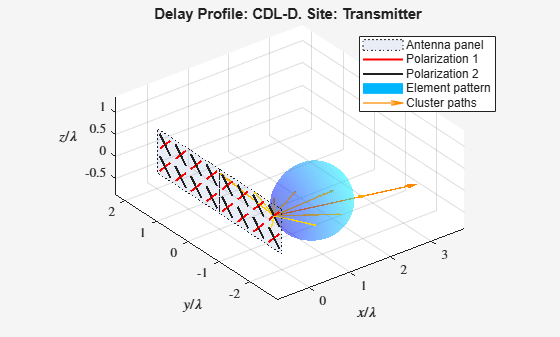`displayChannel(cdl,'LinkEnd','Rx')`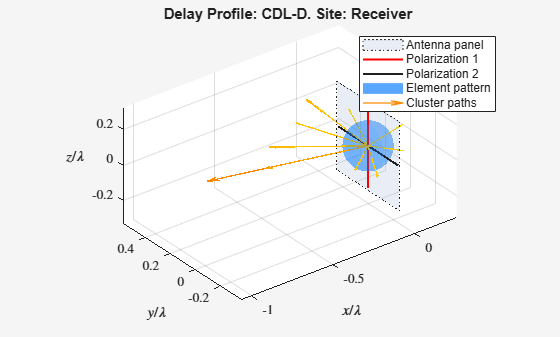Create a random waveform of 1 subframe duration with 8 antennas.

```SR = 15.36e6; T = SR * 1e-3; cdl.SampleRate = SR; cdlinfo = info(cdl); Nt = cdlinfo.NumInputSignals; txWaveform = complex(randn(T,Nt),randn(T,Nt));```

Transmit the input waveform through the channel.

`rxWaveform = cdl(txWaveform);`

Plot channel output and path gain snapshots for various sample density values while using an `nrCDLChannel` System object.

Configure a channel with delay profile CDL-B from TR 38.901 Section 7.7.1. Set the maximum Doppler shift to 300 Hz and the channel sampling rate to 10 kHz.

```cdl = nrCDLChannel; cdl.DelayProfile = 'CDL-B'; cdl.MaximumDopplerShift = 300.0; cdl.SampleRate = 10e3; cdl.Seed = 19;```

Configure the transmit and receive antenna arrays for single-input/single-output (SISO) operation.

```cdl.TransmitAntennaArray.Size = [1 1 1 1 1]; cdl.ReceiveAntennaArray.Size = [1 1 1 1 1];```

Create an input waveform with a length of 40 samples.

```T = 40; in = ones(T,1);```

Plot the step response of the channel (displayed as lines) and the corresponding path gain snapshots (displayed circles) for various values of the `SampleDensity` property. The sample density property controls how often the channel snapshots are taken relative to the Doppler frequency.

• When `SampleDensity` is set to `Inf`, a channel snapshot is taken for every input sample.

• When `SampleDensity` is set to a scalar S, a channel snapshot is taken at a rate of ${\mathit{F}}_{\mathrm{CS}}=2\mathit{S}×\mathit{MaximumDopplerShift}$.

The `nrCDLChannel` object applies the channel snapshots to the input waveform by means of zero-order hold interpolation. The object takes an extra snapshot beyond the end of the input. Some of the final output samples use this extra value to minimize the interpolation error. The channel output contains a transient (and a delay) due to the filters that implement the path delays.

```s = [Inf 5 2]; % sample densities legends = {}; figure; hold on; SR = cdl.SampleRate; for i = 1:length(s) % call channel with chosen sample density release(cdl); cdl.SampleDensity = s(i); [out,pathgains,sampletimes] = cdl(in); chInfo = info(cdl); tau = chInfo.ChannelFilterDelay; % plot channel output against time t = cdl.InitialTime + ((0:(T-1)) - tau).' / SR; h = plot(t,abs(out),'o-'); h.MarkerSize = 2; h.LineWidth = 1.5; desc = ['Sample Density = ' num2str(s(i))]; legends = [legends ['Output, ' desc]]; disp([desc ', Ncs = ' num2str(length(sampletimes))]); % plot path gains against sample times h2 = plot(sampletimes-tau/SR,abs(sum(pathgains,2)),'o'); h2.Color = h.Color; h2.MarkerFaceColor = h.Color; legends = [legends ['Path Gains, ' desc]]; end```
```Sample Density = Inf, Ncs = 40 Sample Density = 5, Ncs = 13 Sample Density = 2, Ncs = 6 ```
```xlabel('Time (s)'); title('Channel Output and Path Gains vs. Sample Density'); ylabel('Channel Magnitude'); legend(legends,'Location','NorthWest');```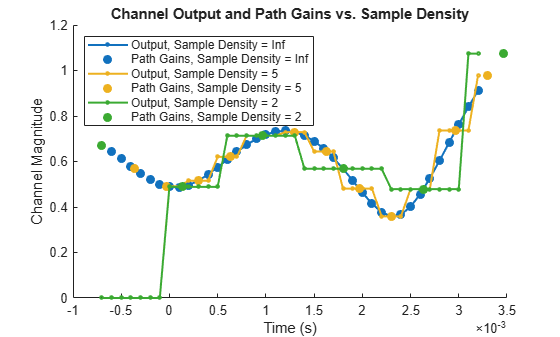Create a CDL channel model. Then specify a light-of-sight (LOS) channel.

```cdl = nrCDLChannel; cdl.DelayProfile = 'CDL-D'; % LOS channel cdl.TransmitAntennaArray.Element = '38.901'; cdl.ReceiveAntennaArray.Element = '38.901';```

Retrieve channel characteristic information. Orient the transmit and receive antenna arrays to point at each other by using the LOS path angles returned in the characteristic information.

```cdlInfo = cdl.info; cdl.TransmitArrayOrientation = [cdlInfo.AnglesAoD(1) cdlInfo.AnglesZoD(1)-90 0]'; cdl.ReceiveArrayOrientation = [cdlInfo.AnglesAoA(1) cdlInfo.AnglesZoA(1)-90 0]';```

Visualize the channel characteristics at the transmitter end.

```cdl.displayChannel('LinkEnd','Tx'); view(0,90)```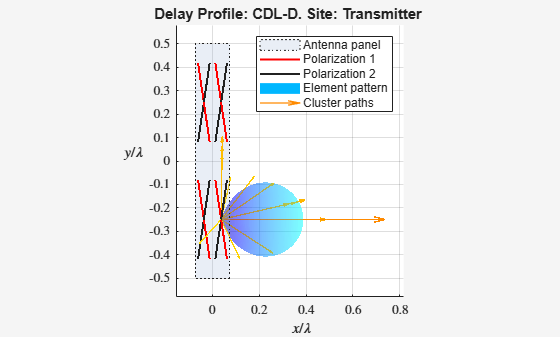Visualize the channel characteristics at the receiver end. The strongest path (LOS) passes through the maximum of the antenna element radiation pattern, which confirms that the antennas point at each other.

```cdl.displayChannel('LinkEnd','Rx') view(0,90)```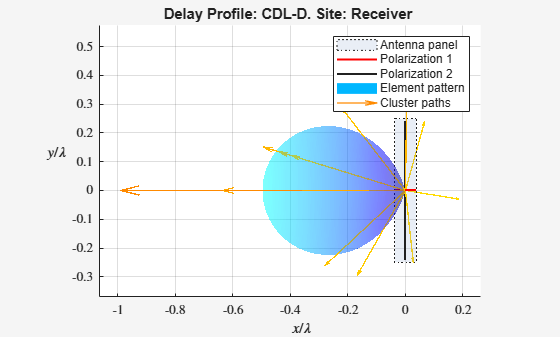Create a CDL channel model. Then specify a phased array for the transmit antenna array.

```cdl = nrCDLChannel; cdl.TransmitAntennaArray = phased.URA;```

Specify a cross-dipole transmit antenna array element to generate circularly polarized fields.

`cdl.TransmitAntennaArray.Element = phased.CrossedDipoleAntennaElement;`

Set the broadside direction of the array toward the positive y-axis. Add a 30 degree downtilt.

```cdl.TransmitAntennaArray.ArrayNormal = 'y'; cdl.TransmitArrayOrientation = [0; 30; 0];```

Set the antenna element spacing to half wavelength.

```lambda = physconst('lightspeed')/cdl.CarrierFrequency; cdl.TransmitAntennaArray.ElementSpacing = [lambda/2 lambda/2];```

Visualize the channel characteristics at the transmitter end.

`cdl.displayChannel('LinkEnd','Tx');`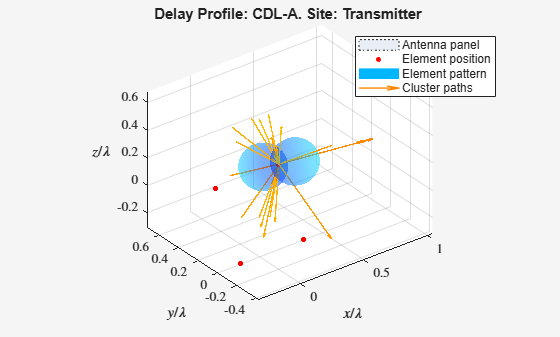3GPP TR 38.901. “Study on channel model for frequencies from 0.5 to 100 GHz.” 3rd Generation Partnership Project; Technical Specification Group Radio Access Network.